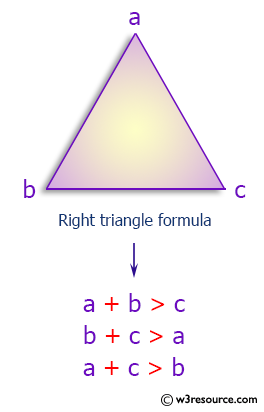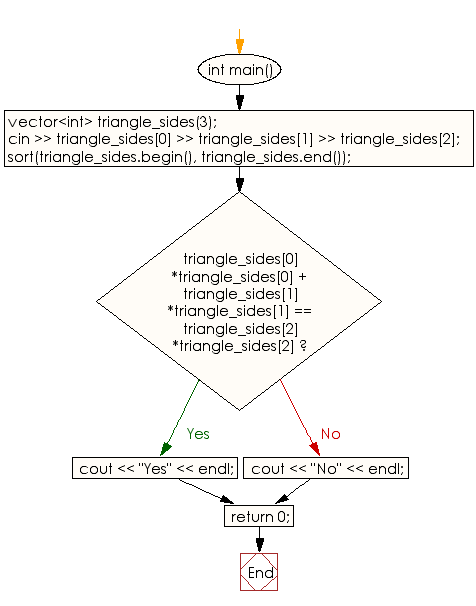﻿ C++ : Check if length of three side form a right triangle

# C++ Exercises: Check whether given length of three side form a right triangle

## C++ Basic: Exercise-65 with Solution

Write a C++ program to check whether a given length of three sides forms a right triangle.

Pictorial Presentation:Sample Solution:

C++ Code :

``````#include <iostream>
#include <vector>
#include <algorithm>
using namespace std;

int main() {

vector<int> triangle_sides(3);

cin >> triangle_sides >> triangle_sides >> triangle_sides;

sort(triangle_sides.begin(), triangle_sides.end());

if (triangle_sides*triangle_sides + triangle_sides*triangle_sides == triangle_sides*triangle_sides)
{
cout << "Yes" << endl;
}
else
{
cout << "No" << endl;
}

return 0;
}
``````

Sample Output:

```Sample input : 6 9 7
Sample Output:
No
```

Flowchart:C++ Code Editor:

What is the difficulty level of this exercise?

﻿

## C++ Programming: Tips of the Day

Why is there no std::stou?

The most pat answer would be that the C library has no corresponding "strtou", and the C++11 string functions are all just thinly veiled wrappers around the C library functions: The std::sto* functions mirror strto*, and the std::to_string functions use sprintf.

Ref: https://bit.ly/3wtz2qA

We are closing our Disqus commenting system for some maintenanace issues. You may write to us at reach[at]yahoo[dot]com or visit us at Facebook